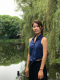Kimberly Liu
3

SAT Subject Test Math 1 vs. Math 2: Which One Should You Take?

Is your SAT score enough to get you into your dream school?

Our free chancing engine takes into consideration your SAT score, in addition to other profile factors, such as GPA and extracurriculars. Create a free account to discover your chances at hundreds of different schools.

There are many decisions you have to make along the college applications journey, and one of these involves the SAT Subject Tests. Not only do you have to decide how many to take, but also which ones to take. For the SAT math subject tests, the decision for which to take is less straightforward than you might imagine. So if you’re considering taking a math subject test, read on to understand which one is better suited for you.

Math 1 and Math 2: Similarities and Differences

In keeping with the math theme of this post, we’ve put together a table illustrating the main similarities and differences between the SAT subjects tests Math Level 1 and Level 2.

 Math 1 Math 2 60 minutes for 50 multiple-choice questions 60 minutes for 50 multiple-choice questions Topics cover those learned in two years of algebra and one year of geometry Topics cover those from two years of algebra and one year of geometry, plus precalculus and trigonometry. Main subjects: Numbers and Operations, Algebra and Functions, Geometry and Measurement, Data Analysis, Statistics and Probability Main subjects: Numbers and Operations, Algebra and Functions, Geometry and Measurement, Data Analysis, Statistics and Probability Topics exclusively covered in Math 1: Plane geometry Focuses on higher-level concepts covered in Math 1, e.g.: trigonometry, vectors, properties of functions Test covers less breadth; questions are often abstract and focus more on depth by requiring multi-step calculations Test covers more topics; questions are more straightforward

Overall, there is a lot of overlap between the two tests.

As part of our free guidance platform, our Admissions Assessment tells you what schools you need to improve your SAT score for and by how much. Sign up to get started today.

How to Decide Between Math 1 and Math 2

One of the first questions you can ask yourself to help decide which test to take is which schools you intend on applying to, and also your potential future major. STEM majors and STEM-focused institutions, such as MIT, Caltech, and Harvey Mudd, often require Math 2.

Even if you don’t plan on pursuing a STEM degree, you may consider taking Math 2 if you have sufficient coursework. Non-standardized classes at different high schools may vary, but a good rule of thumb to follow is if you’ve taken precalculus or trigonometry, you should be able to take Math 2.

If it were simply a question of math level, the question of which math subject test wouldn’t be so difficult. There are various factors of difference: while Math 2 covers more topics than does Math 1, the approach to assessing your knowledge in Math 2 is more straightforward and requires less abstract thinking. Think fewer trick questions, more direct equations.

In terms of grading, Math 2 is also more forgiving. Math 1 has a steeper grading curve than Math 2. This means that to score a full 800 on the test, Math 2 will tolerate more incorrect answers than will Math 1; you may be able to get three questions wrong and still score an 800 for Math 2, but one incorrect answer on Math 1 may prevent you from getting 800.

The scores themselves are important, but remember that for a standardized test such as the SAT, colleges are always stacking you up against your peers (and competitors). A larger percentage of test takers of Math 2 score 800 than those taking Math 1. From the three years between 2016 and 2018, a score of 800 on Math 1 would have put you in the 99th percentile, while the same score of 800 on Math 2 would have put you in the 79th percentile. This means that getting a full score would be impressive for Math 1, but nothing extraordinary for Math 2. This makes sense when we consider that Math 2 generally attracts students who excel in mathematics and who may be aiming to pursue STEM degrees.

If you’re really stuck on deciding which Math Subject Test to take, there’s no better way to see which one is the better choice than to take a practice test of both. If many questions on Math 2 stump you, then you are probably better off taking Math 1; just always be aware of the trend of percentile rankings.

Preparing for the SAT? Download our free guide with our top 8 tips for mastering the SAT.